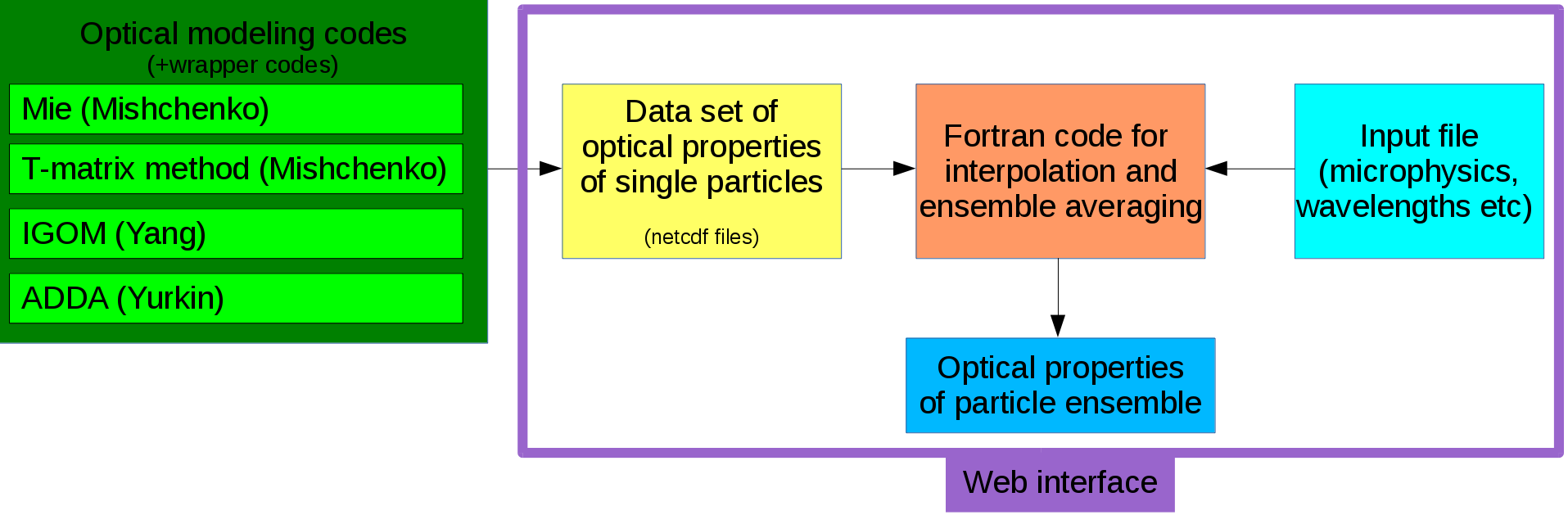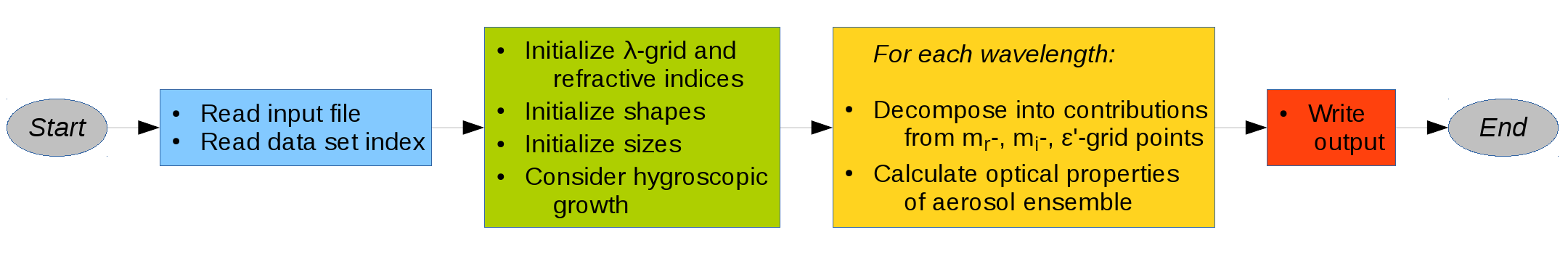back to start page

# Short overview over MOPSMAPThe MOPSMAP package consists of a data set of pre-calculated optical properties of single particles (yellow box) and a corresponding flexible Fortran program (orange box) to calculate the properties of user-defined particle ensembles. The single particle properties have been calculated using the codes listed in the green box. A detailed description is given in a GMD paper.

## Single particle data set

The single particle data set contains the modeled optical properties of spheres and well as spheroids and irregular particles in random orientation. The complete set of optical properties of randomly-oriented particles comprises the extinction efficiency, the scattering efficiency, and expansion coefficients which allow one to calculate the scattering matrix at any scattering angle. The properties were calculated on a grid of size parameters, refractive indices, and shapes, covering wide ranges of these parameters. The modeled data is stored in netcdf files as function of size parameter, thus within each file the refractive index and the particle shape is fixed.

## MOPSMAP Fortran programTo provide the ability to calculate the optical properties of particle ensembles and to interpolate between the grid points of the data set, a user-friendly Fortran program was written. It provides flexibility with respect to how the particles can be mixed.

The user specifies in an ASCII input file the microphysical properties of the ensemble to be calculated as well as some additional quantities like the wavelength. The available options are listed in the MOPSMAP userguide (pdf).

A flow chart of the program is shown above. The MOPSMAP program is started from the command line with the name of the input file as first argument by './mopsmap inputfile.txt'. After reading the input file, the information on the data set, like available shapes, size parameters, and refractive indices, is read by the program. As the next step, the information of wavelengths, refractive indices, shapes, and sizes is prepared for the optical calculations. E.g. all required files are read (e.g. wavelength grid or shape distribution) in this step. Furthermore, if hygroscopic growth was specified in the input file, the microphysical properties of the ensemble are adjusted to represent the 'wet' conditions. Then as the next step, the actual optical calculations are performed wavelength after wavelength. For each wavelength, first the ensemble gets divided into contributions, which correspond to single netcdf files (fixed refractive index and shape) and the size distribution applied to their data. As the second step, the optical properties of each contribution is calculated by integration over the size distribution and added to the sum over all contributions. When the optical properties for all wavelengths are calculated, the results are output to the screen or into files.